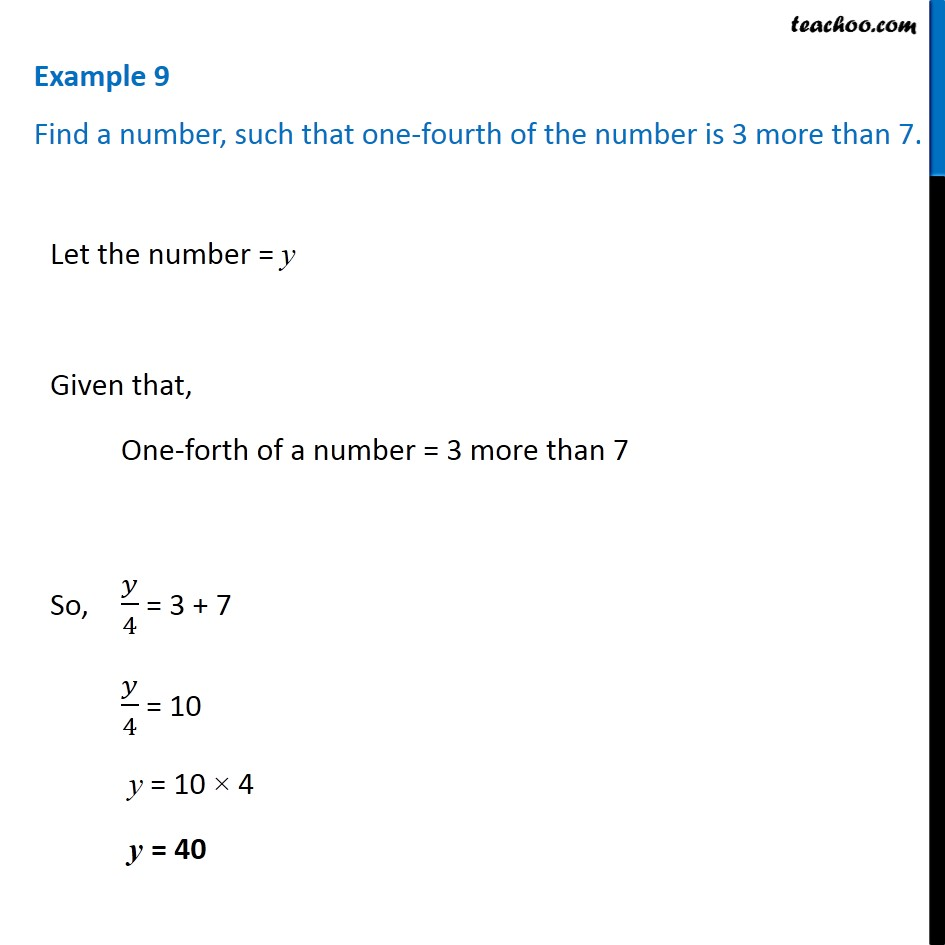Examples

Chapter 4 Class 7 Simple Equations
Serial order wiseLearn in your speed, with individual attention - Teachoo Maths 1-on-1 Class

### Transcript

Example 9 Find a number, such that one-fourth of the number is 3 more than 7. Let the number = y Given that, One-forth of a number = 3 more than 7 So, 𝑦/4 = 3 + 7 𝑦/4 = 10 y = 10 × 4 y = 40